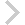FICO Xpress Optimization Examples Repository
 FICO Optimization Community FICO Xpress Optimization HomeRobust network optimization for VPN design

Description
Designing a telecommunication network with uncertain demand, formulated via polyhedral uncertainty sets. This problem is a particular case of a multi-commodity network flow problems, with the important addition of the uncertainty in the traffic demands.

Further explanation of this example: Whitepaper 'Robust Optimization with Xpress', Section 4 Robust network design

Source Files

Data Files

vpn_design.mos

```(!*******************************************************
Mosel Example Problems
======================

file vpn_design.mos
```````````````````
Robust network optimization

(c) 2014 Fair Isaac Corporation
author: P. Belotti, Apr. 2014, rev. Jun. 2015
*******************************************************!)

model "Robust Network"
uses "mmrobust", "mmxprs"

parameters
vpn_data="vpn_data.dat"
end-parameters

declarations
NODES: range                          ! Set of nodes
ARCCOST: dynamic array(NODES, NODES) of real ! Per-unit cost of arc (i,j)

DEM_IN:   array(NODES) of real        ! Total INCOMING demand at each node
DEM_OUT:  array(NODES) of real        ! Total OUTGOING demand at each node

UNITCAP: integer                      ! Per-unit capacity (independent of arc)

NETCOST: linctr                       ! Objective function

! Decision variables
flow: dynamic array(NODES, NODES, NODES, NODES) of mpvar
! flow(i,j,h,k) is 1 iff demand h->k uses arc (i,j)
capacity: dynamic array(NODES, NODES) of mpvar  ! Capacity to install on arc (i,j)

! Uncertain parameters
demand: array(NODES, NODES) of uncertain    ! Uncertain traffic demand
RobustCap: array(NODES, NODES) of robustctr ! Uncertain traffic demand, formulated at each arc

end-declarations

! The following option is necessary to allow uncertain demands to be
! used across multiple capacity constraints

setparam("robust_uncertain_overlap", true)

! Set verbosity level

setparam("xprs_verbose", true)

!**** Data input ****

initializations from vpn_data
NODES ARCCOST
DEM_IN DEM_OUT
UNITCAP
end-initializations

!**** Model formulation ****

forall(i in NODES, j in NODES, h in NODES, k in NODES |
exists(ARCCOST(i,j)) and ARCCOST(i,j) > 0 and h <> k) do
create(flow(i,j,h,k))
flow(i,j,h,k) is_binary
end-do

forall(i in NODES, j in NODES | exists(ARCCOST(i,j)) and ARCCOST(i,j) > 0) do
create(capacity(i,j))
capacity(i,j) is_integer
end-do

! Flow balance at intermediate nodes for each demand(h,k)
forall(i in NODES, h in NODES, k in NODES | i <> h and i <> k and k <> h)
sum(j in NODES | exists(flow(i,j,h,k))) flow(i,j,h,k) -
sum(j in NODES | exists(flow(j,i,h,k))) flow(j,i,h,k) = 0

! Flow balance at source nodes (unnecessary for sink nodes)
forall(i in NODES, h=i, k in NODES | k <> h)
sum(j in NODES | exists(flow(i,j,h,k)) ) flow(i,j,h,k) -
sum(j in NODES | exists(flow(j,i,h,k)) ) flow(j,i,h,k) = 1

! Capacity (robust) constraint

forall(i in NODES, j in NODES | exists(ARCCOST(i,j)) and ARCCOST(i,j) > 0)
RobustCap(i,j) :=
sum(h in NODES, k in NODES | h <> k) demand(h,k) * flow(i,j,h,k) <=
UNITCAP * capacity(i,j)

! Uncertainty set: all demands are nonnegative and constrained by
! total outgoing and incoming demand

forall(h in NODES, k in NODES | h <> k) demand(h,k) >= 0

forall(h in NODES) sum(k in NODES | h <> k) demand(h,k) <= DEM_OUT(h)
forall(h in NODES) sum(k in NODES | h <> k) demand(k,h) <= DEM_IN(h)

! Shortest path length
NetCost := sum(i in NODES, j in NODES | exists(ARCCOST(i,j)) and ARCCOST(i,j) > 0)
ARCCOST(i,j) * capacity(i,j)

!**** Solving ****

minimize(NetCost)

declarations
curNode: integer ! Local variable used in following the path of each demand
end-declarations

! Printing capacities
writeln("Robust solution has total cost ", getobjval)
forall(i in NODES, j in NODES | exists(capacity(i,j)) and capacity(i,j).sol > 0)
writeln("arc ", i, " -- ", j, ": ", capacity(i,j).sol, " units")

writeln("Paths:")

forall(h in NODES, k in NODES | h <> k) do
write("Demand ", h, " --> ", k, ": ")
curNode := h
write(h)
while(curNode <> k) do
forall(j in NODES | flow(curNode, j, h, k).sol > 0.5) do
write(" -- ", j)
curNode := j
end-do
end-do
writeln
end-do

writeln("Active demands at each arc:")

forall(i in NODES, j in NODES | exists(ARCCOST(i,j)) and ARCCOST(i,j) > 0 and
sum(h in NODES, k in NODES) getsol(demand(h,k), RobustCap(i,j)) > 0) do

write("Arc (", i, ",", j, "): ")

forall(h in NODES, k in NODES | getsol(demand(h,k), RobustCap(i,j)) > 0)
write(h, "-->", k, " (", getsol(demand(h,k), RobustCap(i,j)), ");  ")

writeln
end-do
end-model

```